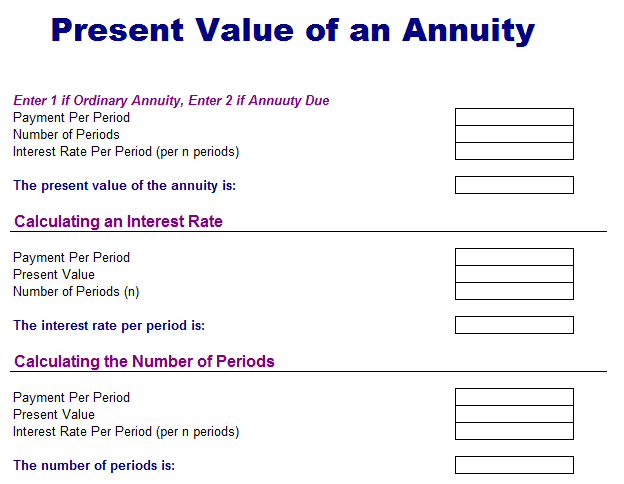# Present Value of Annuity Analysis Template

An Annuity is a type of investment where the investor has to pay or receive money in a set of different intervals. The Sum of money a person receives normally remains constant over the whole life of the annuity. An annuity is considered a stream of cash flows paid out on a monthly or annual basis. You can invest in an annuity through a bank or financial institution after depositing a lump sum payment to the institution in exchange if a series of cash flows are paid out over time. The amount of the lump sum is considered the present value of the annuity. You can easily calculate the amount of payment needed by taking the future value of the annuity. For instance, if you want to invest money for the next 25 years for your retirement get \$150,000 at the time of retirement. You can earn 5 percent interest on the retirement account.

Here is a preview of this free Present Value of Annuity Analysis Template created using MS Excel,### Time Value of Money (TVM)

Financial sayings are different from English or moral proverbs. In finance, there is a famous line about financing, “A bird in the hand is worth more than a bird in the bush”. This is simple to understand that a bird in the bush can easily fly away at any time leaving you empty-handed. The time value of money is an important concept in finance as it will tell you that the value of the dollar can be worth more in the future than today therefore you have to invest at a higher rate of interest than the rate of inflation. The time value of money is based on finance.

### Future Prediction

Every fund manager wants to predict the future before time and it is possible now as you can get calculate the price of a particular stock or bond in the future. It is a certain process to know the investment of your value and fund managers usually forecast the future of markets after considering certain factors that can influence the direction of investments.

### Annuity Factors

The present value of an annuity is calculated by multiplying the cash flows by an annuity factor. The current interest rate and total period for which the investor will receive the cash flow in the future are used as an annuity factor. Generally, the projected interest rate will determine the value of cash flows such as a higher projected interest rate means a high present value of cash flows. Lengthy duration for the cash flows will increase the present value of cash flows.

### Practical Example

Different present value annuity tables will help you to determine the pre-calculated annuity factors. If you want to know the present value of \$500 payments invested at the rate of 8% interest rate in five years, the annuity factor is 19.9636. This shows that the present value of \$500 for 5 years is \$100 x 19.9636 or \$1996.36.  If the same amount is provided for 50 years at the 8% interest rate then the annuity factor will be 61.1674 x \$100 is equal to \$6116.74.